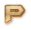# Python Basic Operators Online Test

Instructions:
• Total number of questions : 10.
• Time alloted : 10 minutes.
• Each question carry 1 mark.
• No Negative marks
• DO NOT refresh the page.
• All the best :-)

### What You Will Learn in

This Python Basic Operators Online Test helps you to Boost your Knowledge in Python. In this test, we will cover the topics in the form of questions like

• When both of the operands are the integer then python chops out the fraction part and gives you the round off value, to get the accurate answer use floor division.
• For order of precedence, just remember this PEMDAS where P stands for Parentheses, E stands for Exponential etc.
• Modulus operator gives the remainder.
• % operator gives the remainder of the two dividing numbers.
• We cannot perform a mathematical operation on a string.
• ** have the highest precedence among all operators.